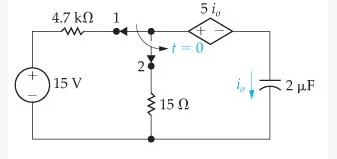# RC circuit where source depends on capacitor current

• Engineering
• miyelmunshi

#### miyelmunshi

Homework Statement
Find Io(t) for t>0 of the circuit shown
Relevant Equations
I(t) = C x dv/dt
V = Q/CI did a kvl loop and got V + 5Io - 15 Io = 0
So, V = 10Io
Then, substituted dq/dt for Io and q/c for V
I get to dq/dt - 50000q = 0
solving this equation i get q = C x e^50000q (c for constant)

my Io = dq/dt
so Io now is 50,000Ce^50000q

now my Q(0) is 3 x 10^-5
So my Io (0) should be, 50,000Ce^1.5

.
I don't know how to find the C now or any of the things i did is even correct

I know the answer should be
15e^-25000t for V(t)
0.75e^-25000t for Io(t)

I don't know how to find the C
See the diagram carefully?

See the diagram carefully?
Ummm can not figure out anything unfortunately :)

See the diagram carefully?
are rest of my process correct ?

See the diagram carefully?
Ok i looked at it again so i can i do a kvl loop for Io(0) and put the voltage of capacitor as 15 v ? then get the value of Io(0) ?

It might be useful to note that the control law for the controlled source is 5*Io and ask yourself what kind of electronic component has the property that the voltage across it is proportional to the current through it. In other words, what standard passive component is the controlled source equivalent to?

••scottdave and berkeman
It might be useful to note that the control law for the controlled source is 5*Io and ask yourself what kind of electronic component has the property that the voltage across it is proportional to the current through it. In other words, what standard passive component is the controlled source equivalent to?
a resistor maybe ? but why is that important ?

You need to be a little more precise about ##i_0##. ##i_0(0)## is ambiguous because there's a discontinuity at ##t=0## in ##i_0##. Can you tell us what ##i_0(0^-)## is, assuming the switch has been in position 1 for a long time?

•scottdave

You need to be a little more precise about ##i_0##. ##i_0(0)## is ambiguous because there's a discontinuity at ##t=0## in ##i_0##. Can you tell us what ##i_0(0^-)## is, assuming the switch has been in position 1 for a long time?
in position 1 as far as i know, no current flows through the circuit as there is no voltage difference, so shouldn't Io be 0 then ? for t < 0 ?

Right. The capacitor acts like an open circuit after it's charged, so ##i_0(0^-)=0## and ##V(0^-)=15~\rm V##.

Instead of focusing on ##i_0##, consider ##Q## for ##t>0##. Recall that the voltage across a capacitor is continuous, so you know that ##V(0^+) = V(0^-) = 15~\rm V##. What does this mean about ##Q(0^+)##?

Right. The capacitor acts like an open circuit after it's charged, so ##i_0(0^-)=0## and ##V(0^-)=15~\rm V##.

Instead of focusing on ##i_0##, consider ##Q## for ##t>0##. Recall that the voltage across a capacitor is continuous, so you know that ##V(0^+) = V(0^-) = 15~\rm V##. What does this mean about ##Q(0^+)##?
i don't really understand what you are getting into but q should be CV so, 3x10^-5 maybe ?

i don't really understand what you are getting into.
Like what part?

but q should be CV so, 3x10^-5 maybe ?
Right, other than the lack of units. So you essentially have an expression for ##q(t)## for ##t>0##, right? From that, you can find the results you want (after you fix the sign mistake).

Like what part?

Right, other than the lack of units. So you essentially have an expression for ##q(t)## for ##t>0##, right? From that, you can find the results you want (after you fix the sign mistake).
um if i fix the sign mistake will the equation be V + 20 Io?

um if i fix the sign mistake will the equation be V + 20 Io?
That's ,not an equation.

Perhaps you mean that ## V = 20 I_0 ## .

a resistor maybe ? but why is that important ?
If the controlled source were replaced with a 5 ohm resistor, would the signs in your equation be any different?

If the controlled source were replaced with a 5 ohm resistor, would the signs in your equation be any different?
i don't think so

That's ,not an equation.

Perhaps you mean that ## V = 20 I_0 ## .
I meant v+20Io = 0